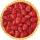Find midpoint

FM=5y+13, MG=5-3y, FG=? M is the midpoint of FG. Use the given information to find the missing measure or value.

Result

x =  16

Solution:Leave us a comment of example and its solution (i.e. if it is still somewhat unclear...):Be the first to comment!To solve this verbal math problem are needed these knowledge from mathematics:

Looking for help with calculating arithmetic mean? Looking for a statistical calculator? Do you have a linear equation or system of equations and looking for its solution? Or do you have quadratic equation?

Next similar examples:

1. MG=7x-15,MG=7x-15, FG=33, x=? Point M is the midpoint of FG. Find unknown x.
2. Midpoint 6FM=8a+1, FG=42, a=? Point M is the midpoint of FG. Find unknown a.
3. Simple equationSolve for x: 3(x + 2) = x - 18
4. Unknown numberIdentify unknown number which 1/5 is 40 greater than one tenth of that number.
5. EquationSolve the equation: 1/2-2/8 = 1/10; Write the result as a decimal number.
6. AverageThe arithmetic mean of the two numbers is 71.7. One number is 5. Calculate the second number.
7. Arithmetic averageThe arithmetic mean of the five numbers is exactly 8. The sum of these four numbers is 30. What is the fifth number?
8. 1.5 divided1.5 divided by 1 = w divided by 4
9. Equation with xSolve the following equation: 2x- (8x + 1) - (x + 2) / 5 = 9
10. CakesGrandmother baked cakes. Half of its was poppy, quarter with plum jam and 16 cheesecakes. How many cakes she baked in total?
11. Equation with fractionsSolve equation: ? It is equation with fractions.
12. Cleaning windowsCleaning company has to wash all the windows of the school. The first day washes one-sixth of the windows of the school, the next day three more windows than the first day and the remaining 18 windows washes on the third day. Calculate how many windows ha
13. Two trainsThere were 159 freight wagons on the railway station creating 2 trains. One had 15 more wagons than the other. How many wagons did each train have?
14. GardenThe garden around the new majer is divided as follows: 35% vegetable, 30% fruit orchards, 10% flowers and the remaining 120 m2 are lawns. What is the total area of the garden?
15. Strange xFor what x is true ??
16. FactoryIn the factory workers work in three shifts. In the first inning operates half of all employees in the second inning and a third in the third inning 200 employees. How many employees work at the factory?
17. Percentage - fractionsAbout what percentage we must increase number 1/6 to get number 1/3?